# Non Verbal Analogy Questions and Answers### Types of Analogy:

Semantic: A semantic analogy involves word meanings, and they might ask a student to recognize synonyms, antonyms, word intensity or parts of an expression.

Classification: A classification analogy tests relationships having to do with categories or parts of a whole.

Association: Association analogies might involve relationships such as characteristics of an object, order, cause and effect, function of an object, or tools used for a purpose.

Non-semantic: Non-semantic analogies involve logic or mathematics, and might test a student’s knowledge of fractions, multiples or negation. They could also contain letter or sound relationships, such as sound patterns, rhymes or homophones.

### Non Verbal Analogy Questions and Answers:

Each of the following questions consists of two sets of figures. Figures A, B, C and D constitute the Problem Set while figures 1, 2, 3, 4 and 5 constitute the Answer Set. There is a definite relationship between figures A and B. Establish a similar relationship between figures C and D by selecting a suitable figure from the Answer Set that would replace the question mark (?) in fig. (D).

Select a suitable figure from the Answer Figures that would replace the question mark (?).

Related: Profit and Loss Problems

Question:
Total numbers of triangles in below picture?(1) 20
(2) 24
(3) 28
(4) 32

Question:
From the given answer figures, select the one in which the question figure is hidden/embedded.
Question Figure: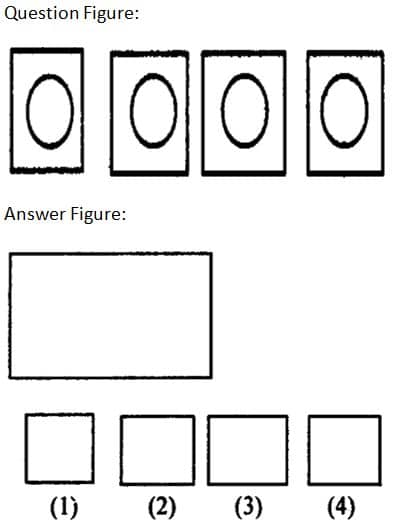Which of the following five Answer Figures on the right should come after the Problem Figures on the left, if the sequence were continued?

Question: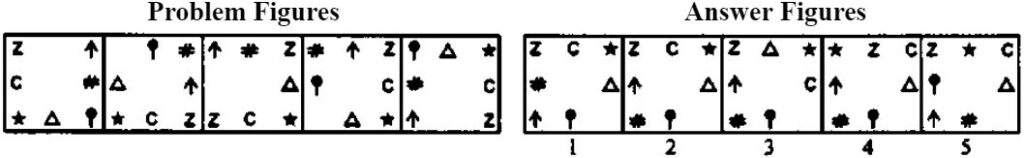Related: Logarithm questions

Question: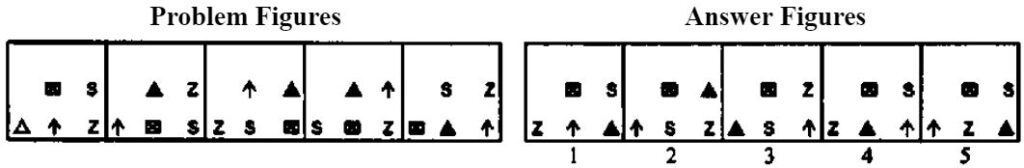Question: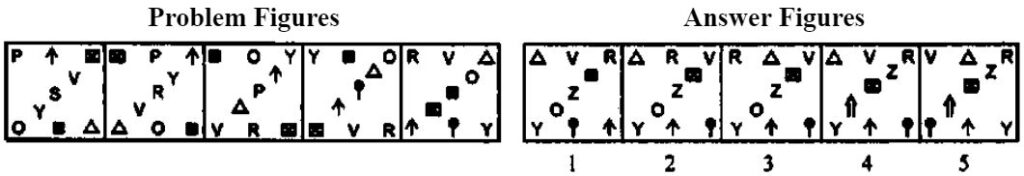Question: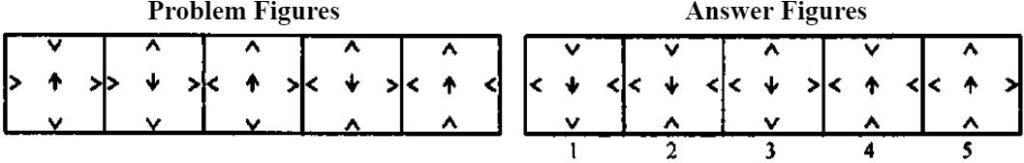Question: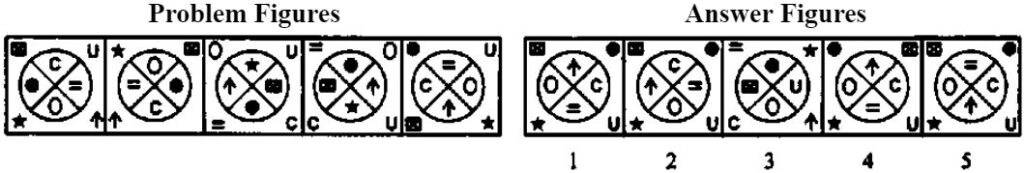Related: English writing Symbols

Question: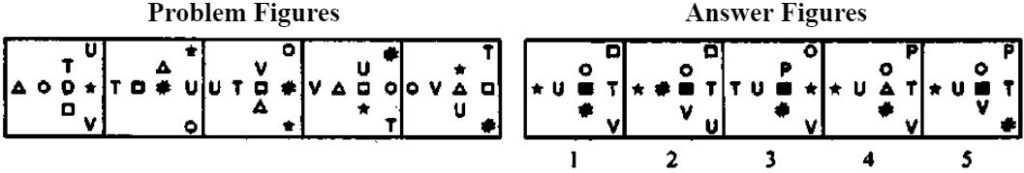#### Yash Bansal

Yash is co-founder of Examsegg. Yash loves to solve questions on reasoning and mathematics. He taught himself how to blend different techniques to get accurate solution in lesser time.

• Chanchal says:

If the population of a certain country is 120,256,000 and its land area is 2,998,000 square kilometers, then the population per square kilometer is closest to which of the following?
A. 4
B. 6
C. 20
D. 40

• Namish says:

Good questions for practice

• Ramesh prakash says:

I mever understood non verbal reasoning questions